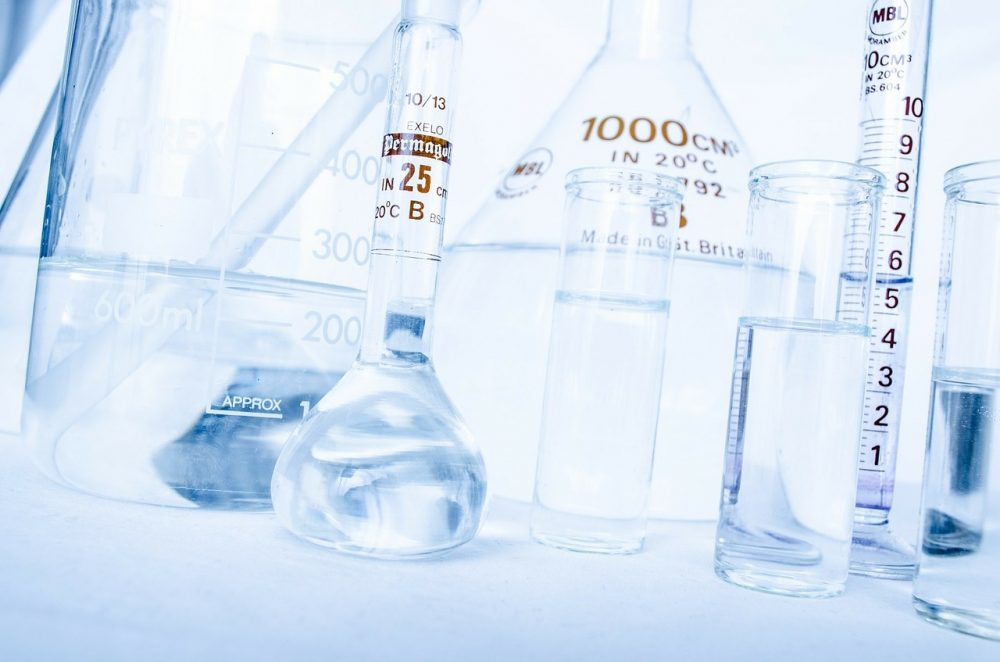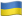# What Is Kw In Chemistry? Understanding Autoionization Of Water

In chemistry, water is the most common solvent for both basic and acidic reactions. It is referred to as amphoteric because it is both an acid and base. From this fact, one question that students ask is: “What happens when water molecules react with other water molecules?” It results in self ionization, Kw. In this post, we will go deeper into the concept to establish what is Kw in chemistry and how to calculate it.## What Does Kw Mean In Chemistry?

Water contains both acidic and basic molecules. Because acids and bases will always react when put together, it simply means that water will react with itself! This sounds very strange. But in reality, it actually happens. The water molecules exchange protons in a process referred to as autoionization of water. This process can be expressed in the following equation (a) below:

H2​O(l)+H2​O(l)⇌H3​O+(aq)+OH−(aq)

In the above equation, one water molecule can be seen donating a proton and, therefore, acts as a Bronsted-Lowry acid. Then, another molecule accepts the molecule and, therefore, acts as a Bronsted-Lowry base. After the reaction, two molecules are formed, Hydronium ions and hydroxide ions. This reaction takes place all the time in any quantity of water.

If you have a sample of pure water, it means that the concentration of Hydronium ions and hydroxide ions is equal. Here is a demonstration in an equation (call it equation B).

In pure water: [H3​O+]=[OH−]

It is important to note that the process demonstrated in the equation above is easily reversible because water is a weak base and a weak acid. To establish the concentrations, it is important to look at the next concept of the autoionization constant.

### Closer Look At Kw Autoionization Of Water

Need to know exactly what is the equation for autoionization of water? Autoionization constant is expressed as demonstrated in equation  (C) below.

Kw ​= [H3​O+][OH−]

At this point, it is prudent to appreciate that when writing Kw constant chemistry expressions, you omit the concentrations. What does this imply when writing or working on Kw? It means that Kw, in this case, is a pure water sample.

Note that Kw in chemistry is dependent on temperature. For example, you can calculate the Kw chemistry equation value at 250C utilizing H3​O+, which is closely related to water PH. At 25 degrees, the PH of pure water is 7. Therefore, what is the concentration of hydronium ions in such a sample? Here is a demonstration in equation (D):

At 250C [H3​O+]=10−pH=10−7 M

So, how to find Kw chemistry? Based on college homework helper experts to understand chemistry Kw, we know that the concentration of hydroxide and hydronium ions follows the ration of 1:1 in the autoionization of water equation. Therefore, we can advance equation (D) above to calculate hydroxide ions, as demonstrated in equation (E) below:

At 250C [OH−]=[H3​O+]=10−7 M

In many cases, students find it hard to visualize the entire reaction. However, 10-7 is a very small figure, and, therefore, only a relatively small quantity of water will be in the ionized form. From equation (E) above, we can go ahead and calculate Kw of water at 250C. See the equation (E) of how to calculate Kw chemistry below:

Kw​=(10−7)×(10−7)=10−14 at 25∘C

### Kw Of Water And Temperature Changes

One thing you need to appreciate when looking at Kw definition chemistry and Kw constant is that the PH varies depending on temperatures. Using the equation (A), the first equation in this post, a forward reaction absorbs heat.

H2​O(l)+H2​O(l)⇌H3​O+(aq)+OH−(aq)

Using Le Chatelier’s Principle, you will find that raising the temperature of water results in the equilibrium working to lower the temperature. This is achieved through heat absorption. It also implies that forward reaction is going to be favored (more hydronium and hydroxide ions will be created.) The overall impact will be lowering of Kw as the temperature moves up. To demonstrate the changes to Kw as temperature changes, have a look at the table below:

 T (°C) Kw (mol2 dm-6) pH 0 0.114 x 10-14 7.47 10 0.293 x 10-14 7.27 20 0.681 x 10-14 7.08 25 1.008 x 10-14 7.00 30 1.471 x 10-14 6.92 40 2.916 x 10-14 6.77 50 5.476 x 10-14 6.63 100 51.3 x 10-14 6.14

Take a closer look at the Kw values, and you will realize that the value is actually moving down as the temperature increases. When learning about self-ionization, h+ definition, and how to calculate Kw chemistry, it is important to be cautious. Although Kw is changing as the temperature changes, the water remains neutral. This is caused by the fact that both hydrogen ions and hydroxide ions change in the same proportion.

### Kw In Chemistry …. Make It Fun And Simple

For many students in college, understanding Kw chemistry concepts such as what does h+ mean in chemistry and how to calculate Kw chemistry is never easy. To find calculating Kw value simple and fun, you should go back to the basics of what acids and bases are. Then, work your way up by exploring how the two apply in water. At every moment, when calculating the value of Kw water, you should note that you are dealing with pure water. Hopefully now you understand what is Kw chemistry.

If you find the subject still hard, you should not give up. For example, if getting the Kw value for the question you have been given by the tutor is an uphill task because of a tight deadline, or the concept is hard, you can always seek cheap online writing help and reliable chemistry homework online help. Homework help for students assists them in completing difficult tasks so that they can engage in other activities, beat tight deadlines, or develop important skills.

Do not let Kw value calculation stress you, use this post, and seek help to become a pro!

Let's stand with the heroesAs Putin continues killing civilians, bombing kindergartens, and threatening WWIII, Ukraine fights for the world's peaceful future.

Donate Directly to Ukraine